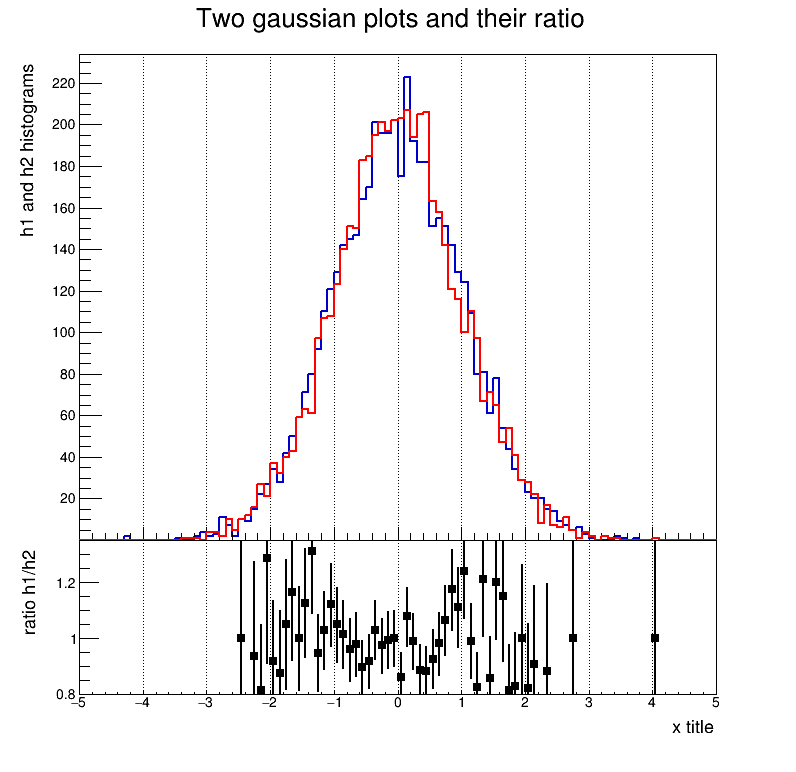# Ratioplot¶

Display two histograms and their ratio.

This program illustrates how to plot two histograms and their ratio on the same canvas. Original macro by Olivier Couet.

Author: Michael Moran
This notebook tutorial was automatically generated with ROOTBOOK-izer from the macro found in the ROOT repository on Thursday, June 04, 2020 at 02:47 AM.

In :
from ROOT import TCanvas, TColor, TGaxis, TH1F, TPad
from ROOT import kBlack, kBlue, kRed

def createH1():
h1 = TH1F("h1", ("Two gaussian plots and their ratio; x title; h1 and h2"
" histograms"), 100, -5, 5)
h1.SetLineColor(kBlue+1)
h1.SetLineWidth(2)
h1.FillRandom("gaus")
h1.GetYaxis().SetTitleSize(20)
h1.GetYaxis().SetTitleFont(43)
h1.GetYaxis().SetTitleOffset(1.55)
h1.SetStats(0)
return h1

def createH2():
h2 = TH1F("h2", "h2", 100, -5, 5)
h2.FillRandom("gaus")
h2.SetLineColor(kRed)
h2.SetLineWidth(2)
return h2

def createRatio(h1, h2):
h3 = h1.Clone("h3")
h3.SetLineColor(kBlack)
h3.SetMarkerStyle(21)
h3.SetTitle("")
h3.SetMinimum(0.8)
h3.SetMaximum(1.35)
# Set up plot for markers and errors
h3.Sumw2()
h3.SetStats(0)
h3.Divide(h2)

y = h3.GetYaxis()
y.SetTitle("ratio h1/h2 ")
y.SetNdivisions(505)
y.SetTitleSize(20)
y.SetTitleFont(43)
y.SetTitleOffset(1.55)
y.SetLabelFont(43)
y.SetLabelSize(15)

x = h3.GetXaxis()
x.SetTitleSize(20)
x.SetTitleFont(43)
x.SetTitleOffset(4.0)
x.SetLabelFont(43)
x.SetLabelSize(15)

return h3

c = TCanvas("c", "canvas", 800, 800)
# Upper histogram plot is pad1
pad1.SetBottomMargin(0)  # joins upper and lower plot
# Lower ratio plot is pad2
c.cd()  # returns to main canvas before defining pad2
pad2.SetTopMargin(0)  # joins upper and lower plot


Welcome to JupyROOT 6.23/01


create required parts

In :
h1 = createH1()
h2 = createH2()
h3 = createRatio(h1, h2)


draw everything

In :
pad1.cd()
h1.Draw()
h2.Draw("same")


to avoid clipping the bottom zero, redraw a small axis

In :
h1.GetYaxis().SetLabelSize(0.0)
axis = TGaxis(-5, 20, -5, 220, 20, 220, 510, "")
axis.SetLabelFont(43)
axis.SetLabelSize(15)
axis.Draw()

from ROOT import gROOT